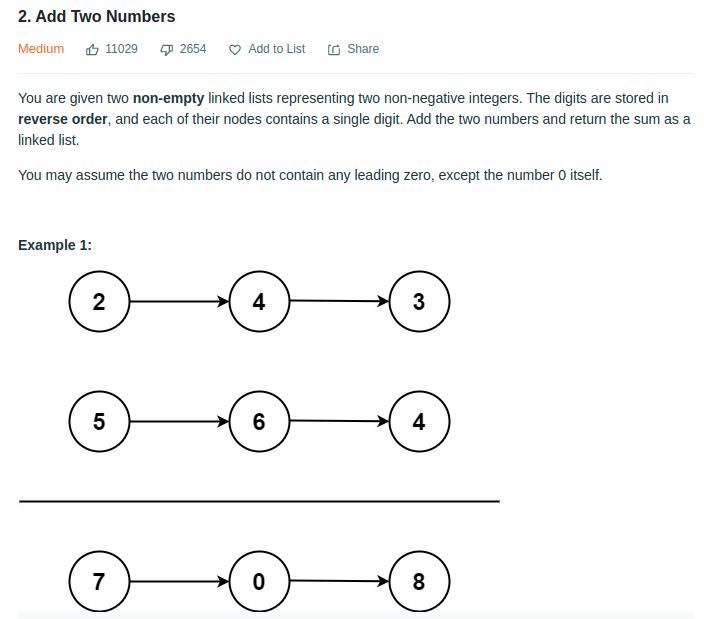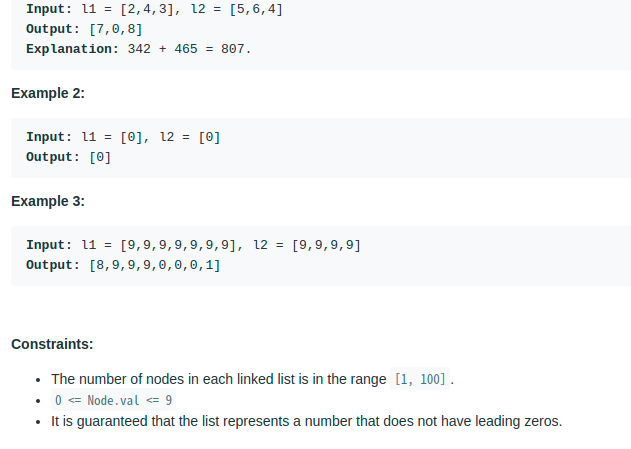# [Leetcode - Algorithm] Add two Numbers

hareguu·2021년 3월 7일
0

## leetcode

목록 보기
3/3

### Questionvar addTwoNumbers = function (l1, l2) {
let carry = 0;
while (l1 || l2) {
let l1Value = l1? l1.val : 0;
let l2Value = l2? l2.val : 0;
let sum = l1Value + l2Value + carry;
carry = 0;
let newValue = sum;
if (sum > 9) {
newValue = sum % 10;
carry = 1;
}
node.next = new ListNode(newValue);
node = node.next;
if (l1) {
l1 = l1.next;
}
if (l2) {
l2 = l2.next;
}
}
if (carry) {
node.next = new ListNode(carry);
}
};